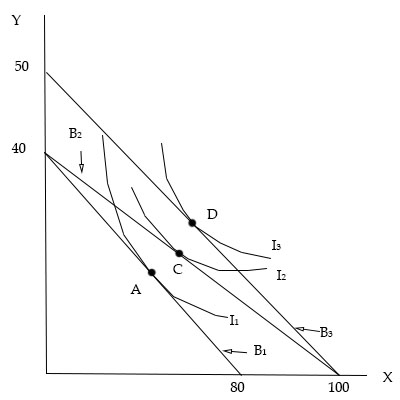# The price of good X is $50. a) What is the consumer's income on budget line B_1? b) What is the... ## Question: The price of good {eq}X {/eq} is {eq}$50 {/eq}.

a) What is the consumer's income on budget line {eq}B_1 {/eq}?

b) What is the price of good {eq}Y {/eq} on Budget line {eq}B_1 {/eq}?

c) What is the equation of the budget line {eq}B_1 {/eq}, given the prices and income that you have determined?

d) What is the consumer's marginal rate of substitution when she is at equilibrium at point {eq}A {/eq}?

e) What are the prices of {eq}X {/eq} and {eq}Y {/eq} on budget line {eq}B_2 {/eq}? You may assume that the income is the same as budget line {eq}B_1 {/eq}.

f) What are the prices of {eq}X {/eq} and {eq}Y {/eq} on budget line {eq}B_3 {/eq}? You may assume that the income is the same as budget line {eq}B_1 {/eq}.

g) Assume that the prices remain at the levels indicated by {eq}B_1 {/eq}. However, income increases such that the budget line changes from {eq}B_1 {/eq} to {eq}B_3 {/eq}. What is the income level at {eq}B_3 {/eq}?## Budget:

A budget is a very important factor for the consumer in the market because it decides the consumer's purchasing power then. The budget or the purchasing power of consumers increases either the fall in price or the increase in income.

a)

The consumer's income on budget line B1 is calculated below:

{eq}\begin{align*} {P_X} &= \50\\ Income &= 50 \times 80\\ &= 4000 \end{align*} {/eq} The income of the consumer will be4000.

b)

The price of good Y on Budget line B1 is calculated below:

{eq}\begin{align*} {P_Y} &= \dfrac{{4000}}{{40}}\\ &= 100 \end{align*} {/eq}

The price of good Y will be 100. c) The equation of the budget line B1 is calculated below: {eq}\begin{align*} {P_X} &= \ 50\\ {P_Y} &= \100\\ M &= 4000\\ {P_X}X + {P_Y}Y &= M\\ 50X + 100Y &= 4000 \end{align*} {/eq} d) The consumer's marginal rate of substitution when she is at equilibrium at point A is calculated below: {eq}\begin{align*} MR{S_{XY}} &= \dfrac{{{P_X}}}{{{P_Y}}}\\ &= \dfrac{{50}}{{100}}\\ &= \dfrac{1}{2} \end{align*} {/eq} e) The prices of X and Y on budget line B2 are calculated below: {eq}\begin{align*} M &= 4000\\ X &= 100\\ Y &= 40\\ {P_X}X + {P_Y}Y &= M\\ 100{P_X} + 40{P_Y} &= 4000\\ {P_X} &= \dfrac{{4000}}{{100}}\\ &= 40\\ {P_Y} &= \dfrac{{4000}}{{40}}\\ &= 100 \end{align*} {/eq} The price of good X will be40, and good Y will be 100. f) The prices of X and Y on budget line B3 are calculated below: {eq}\begin{align*} M &= 4000\\ X &= 100\\ Y &= 50\\ {P_X}X + {P_Y}Y &= M\\ 100{P_X} + 50{P_Y} &= 4000\\ {P_X} &= \dfrac{{4000}}{{100}}\\ &= 40\\ {P_Y} &= \dfrac{{4000}}{{50}}\\ &= 80 \end{align*} {/eq} The price of good X will be40, and good Y will be 80. g) The income level at B3 is calculated below: {eq}\begin{align*} X &= 100\\ {P_X} &= 50\\ Income &= X \times {P_X}\\ &= 100 \times 50\\ &= 5000 \end{align*} {/eq} The income level will be5000.